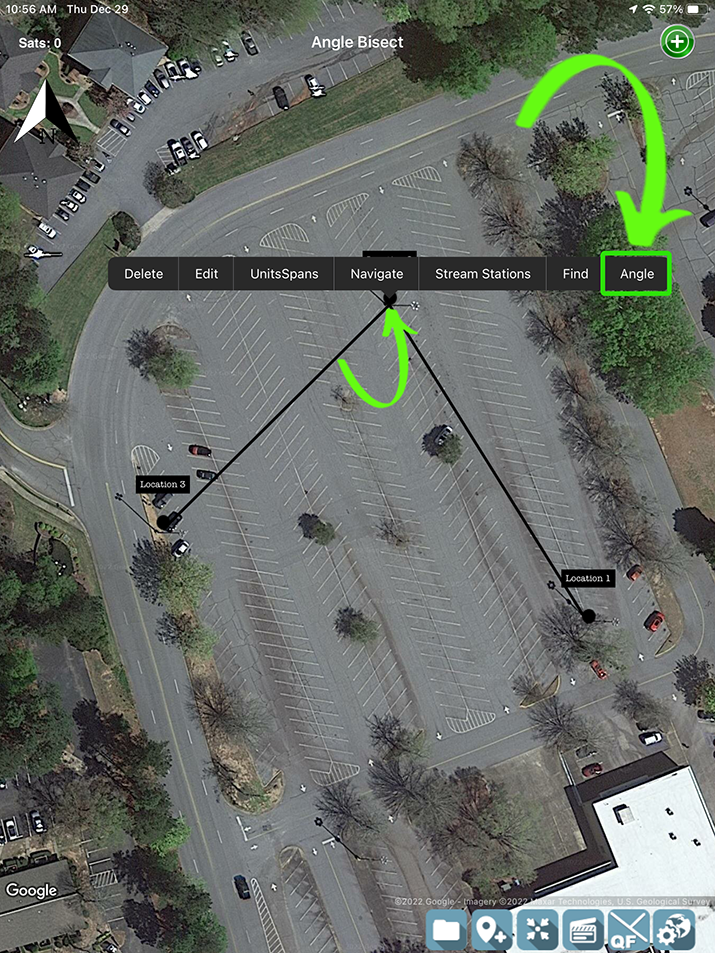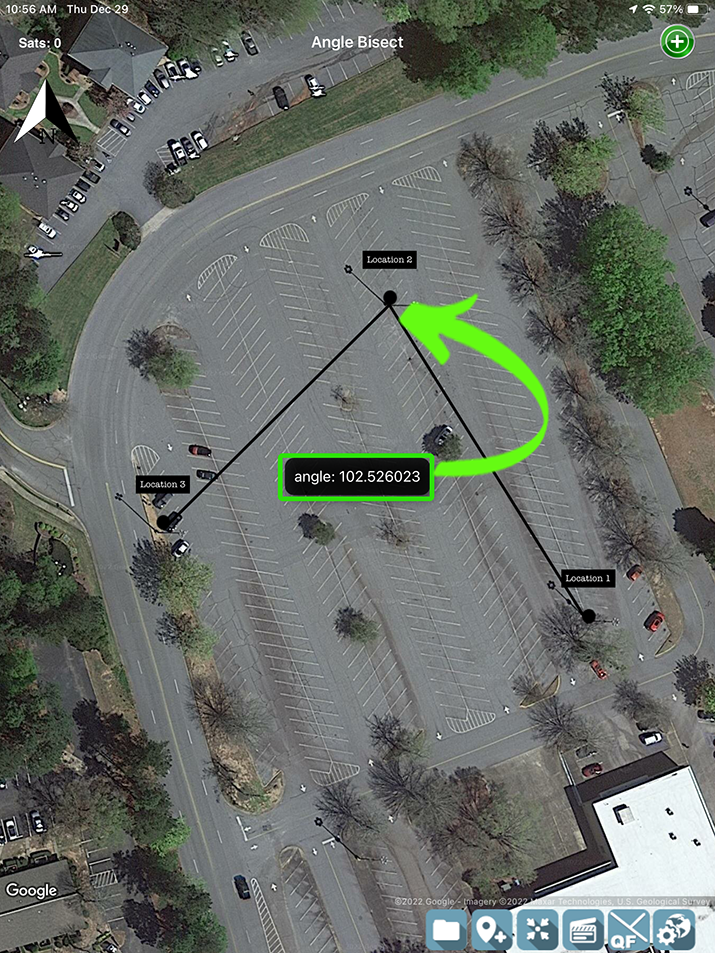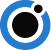# Get Angle

## Get Angle

Get Angle is a function in Orbitas that allows you to calculate the angle of two intersecting lines.

#### Calculating Angle:

To calculate your angle of two collected intersecting lines simply click on the middle point of the line you would like to calculate an angle for.

Select ANGLE from the Location Quick Menu.

*This function requires wi-fi or cell data connectivity to calculate.Back to Orbitas Support